# Examples¶

BurnMan comes with a large collection of example programs under examples/. Below you can find a summary of the different examples. They are grouped into three categories: Class examples, Simple Examples and More Advanced Examples. The Class examples introduce the main class types in BurnMan, and covers similar ground to The BurnMan Tutorial but in a little more detail. Simple Examples provides introductions to some of the seismic and chemical functions. More Advanced Examples covers functionality which is more suited to research projects.

Finally, we also include the scripts that were used for all computations and figures in the 2014 BurnMan paper in the misc/ folder, see Reproducing Cottaar, Heister, Rose and Unterborn (2014).

## Class examples¶

The following is a list of examples that introduce the main classes of BurnMan objects:

### example_mineral¶

This example shows how to create mineral objects in BurnMan, and how to output their thermodynamic and thermoelastic quantities.

Mineral objects are the building blocks for more complex objects in BurnMan. These objects are intended to represent minerals (or melts, or fluids) of fixed composition, with a well defined equation of state that defines the relationship between the current state (pressure and temperature) of the mineral and its thermodynamic potentials and derivatives (such as volume and entropy).

Mineral objects are initialized with a dictionary containing all of the parameters required by the desired equation of state. BurnMan contains implementations of may equations of state (Equations of state).

Uses:

Demonstrates:

• Different ways to define an endmember

• How to set state

• How to output thermodynamic and thermoelastic properties

Resulting figure: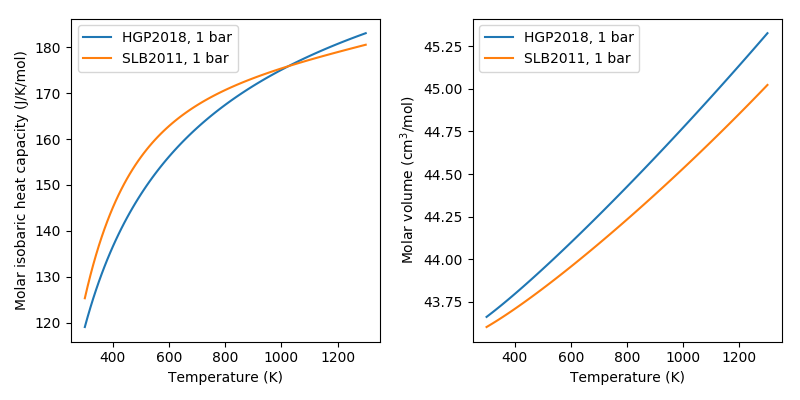### example_gibbs_modifiers¶

This example script demonstrates the modifications to the gibbs free energy (and derivatives) that can be applied as masks over the results from the equations of state.

These modifications currently take the forms:

• Landau corrections (implementations of Putnis (1992) and Holland and Powell (2011)

• Bragg-Williams corrections (implementation of Holland and Powell (1996))

• Linear (a simple delta_E + delta_V*P - delta_S*T

• Magnetic (Chin, Hertzman and Sundman (1987))

Uses:

Demonstrates:

• creating a mineral with excess contributions

• calculating thermodynamic properties

Resulting figures: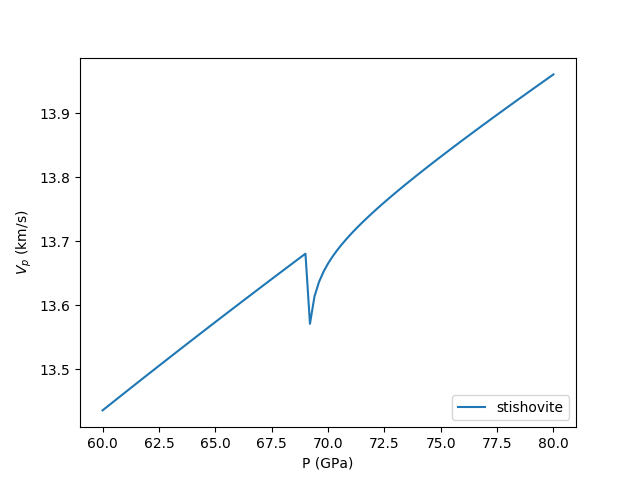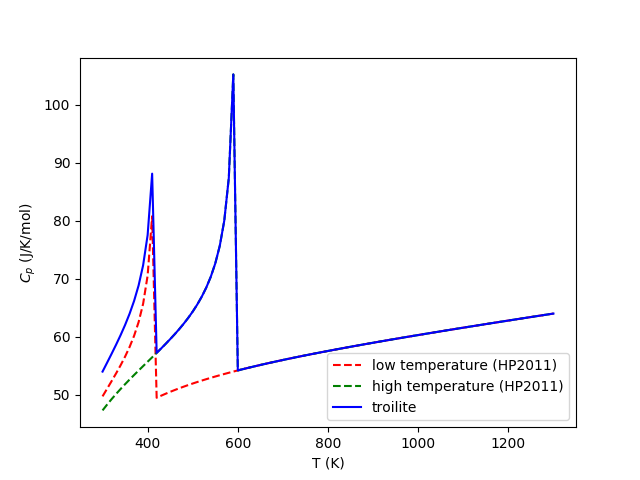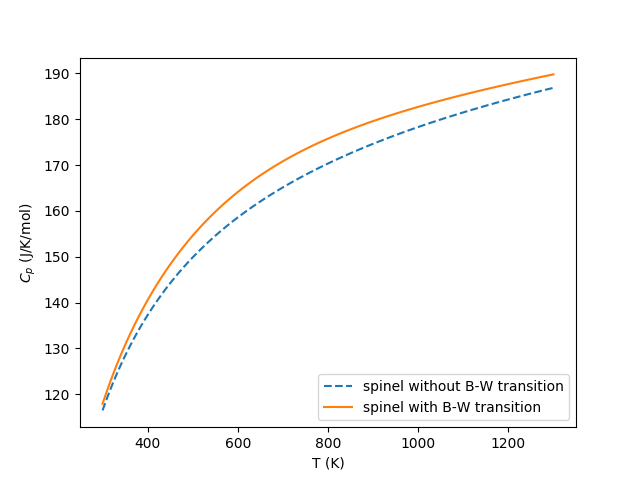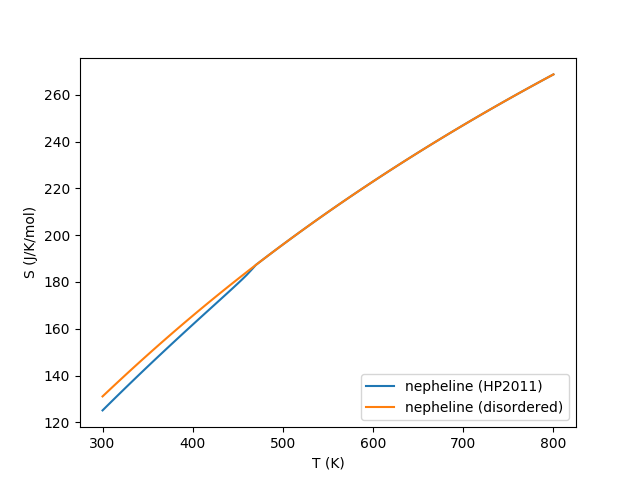### example_solution¶

This example shows how to create different solution models and output thermodynamic and thermoelastic quantities.

There are four main types of solution currently implemented in BurnMan:

1. Ideal solutions

2. Symmmetric solutions

3. Asymmetric solutions

4. Subregular solutions

These solutions can potentially deal with:

• Disordered endmembers (more than one element on a crystallographic site)

• Site vacancies

• More than one valence/spin state of the same element on a site

Uses:

Demonstrates:

• Different ways to define a solution

• How to set composition and state

• How to output thermodynamic and thermoelastic properties

Resulting figures: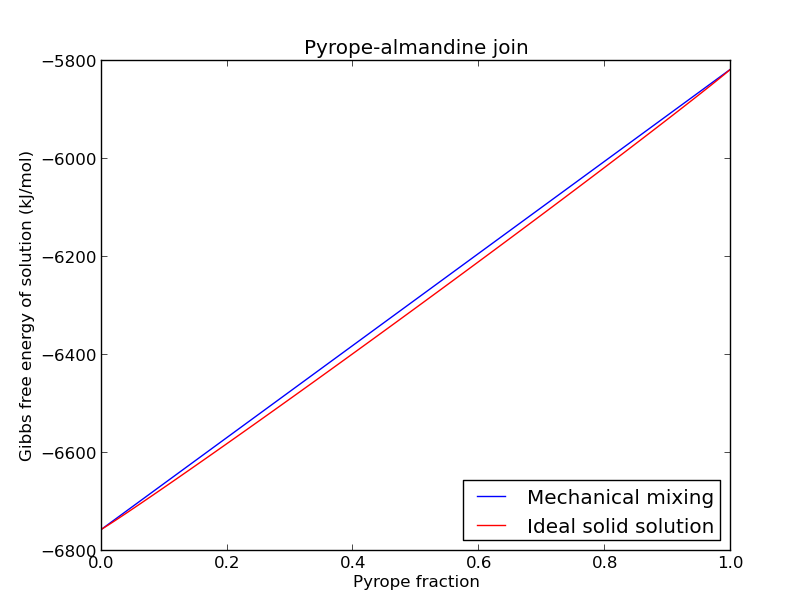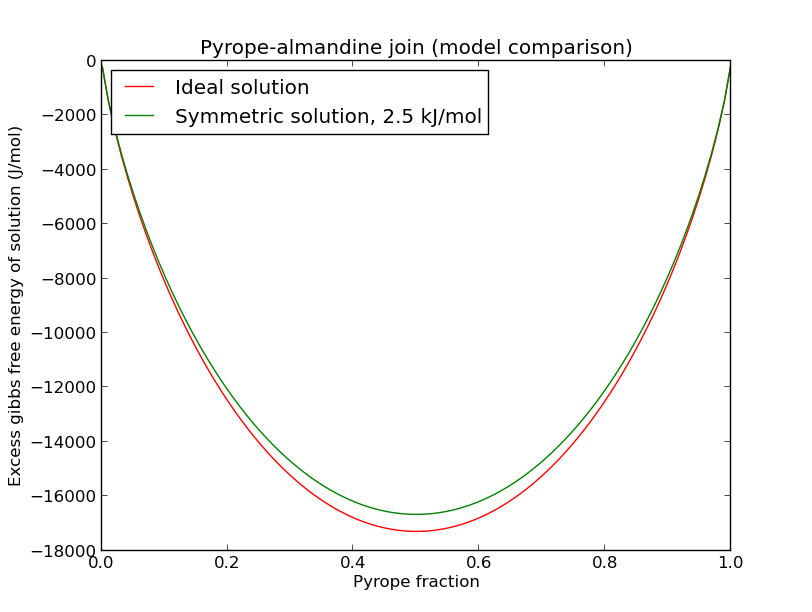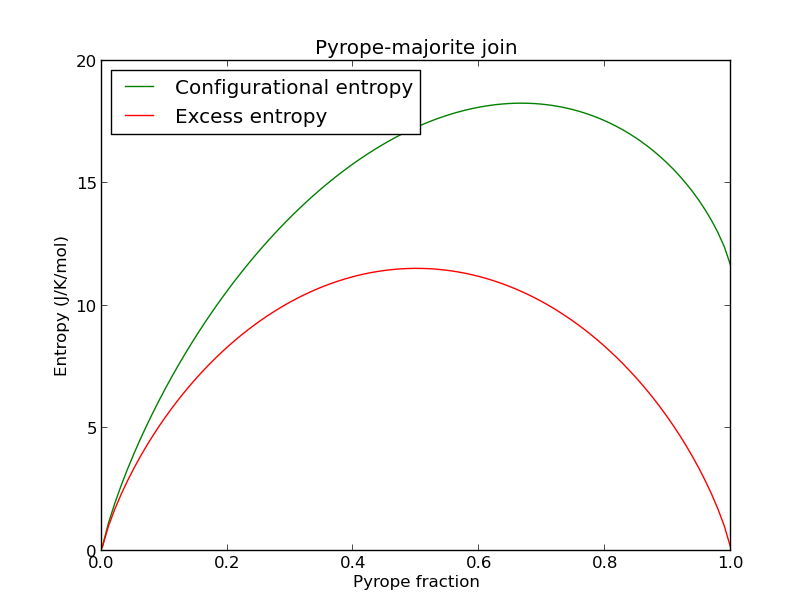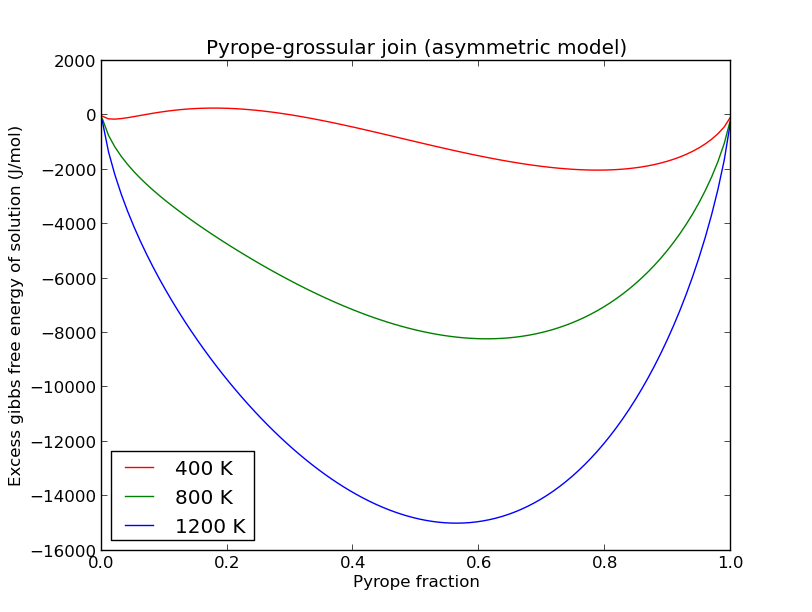### example_composite¶

This example demonstrates the functionalities of the burnman.Composite class.

Uses:

Demonstrates:

• How to initialize a composite object containing minerals and solutions

• How to set state and composition of composite objects

• How to interrogate composite objects for their compositional, thermodynamic and thermoelastic properties.

• How to use the stoichiometric and reaction affinity methods to solve simple thermodynamic equilibrium problems.

Resulting figures: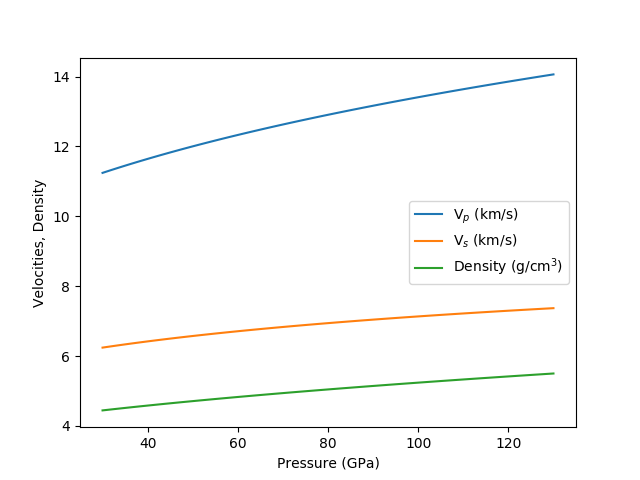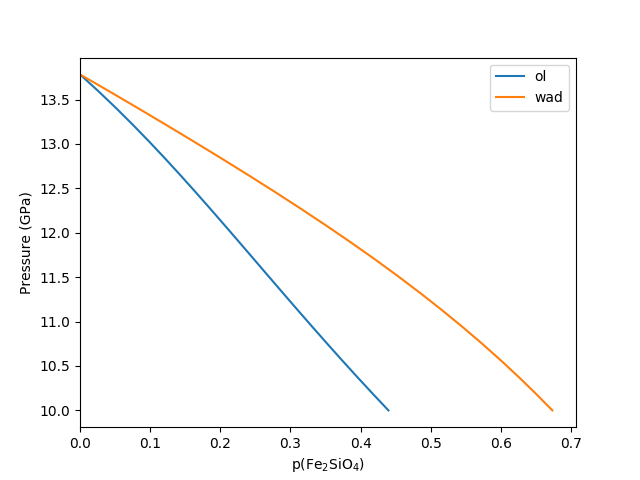### example_mineral¶

This example shows how to create mineral objects in BurnMan, and how to output their thermodynamic and thermoelastic quantities.

Mineral objects are the building blocks for more complex objects in BurnMan. These objects are intended to represent minerals (or melts, or fluids) of fixed composition, with a well defined equation of state that defines the relationship between the current state (pressure and temperature) of the mineral and its thermodynamic potentials and derivatives (such as volume and entropy).

Mineral objects are initialized with a dictionary containing all of the parameters required by the desired equation of state. BurnMan contains implementations of may equations of state (Equations of state).

Uses:

Demonstrates:

• Different ways to define an endmember

• How to set state

• How to output thermodynamic and thermoelastic properties

### example_calibrants¶

This example demonstrates the use of BurnMan’s library of pressure calibrants. These calibrants are stripped-down versions of BurnMan’s minerals, in that they are only designed to return pressure as a function of volume and temperature or volume as a function of pressure and temperature.

Uses:

Demonstrates:

• Use of the Calibrant class

• Conversion between pressures given by two different calibrations.

### example_anisotropy¶

This example illustrates the basic functions required to convert an elastic stiffness tensor into elastic properties.

Specifically uses:

Demonstrates:

• anisotropic functions

Resulting figure:### example_anisotropic_mineral¶

This example illustrates how to create and interrogate an AnisotropicMineral object.

Specifically uses:

Demonstrates:

• anisotropic functions

Resulting figure: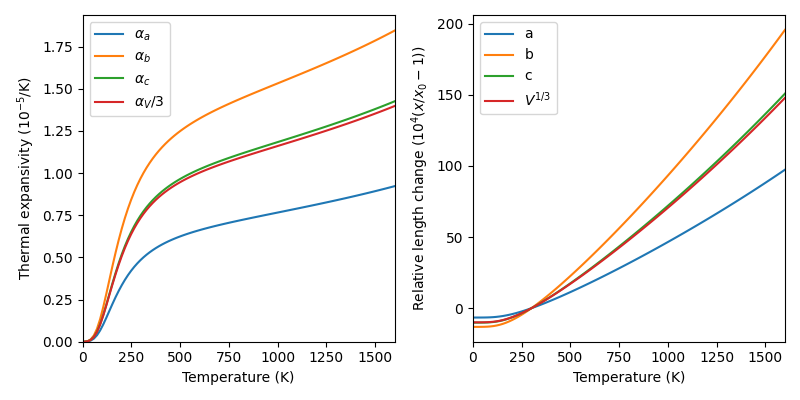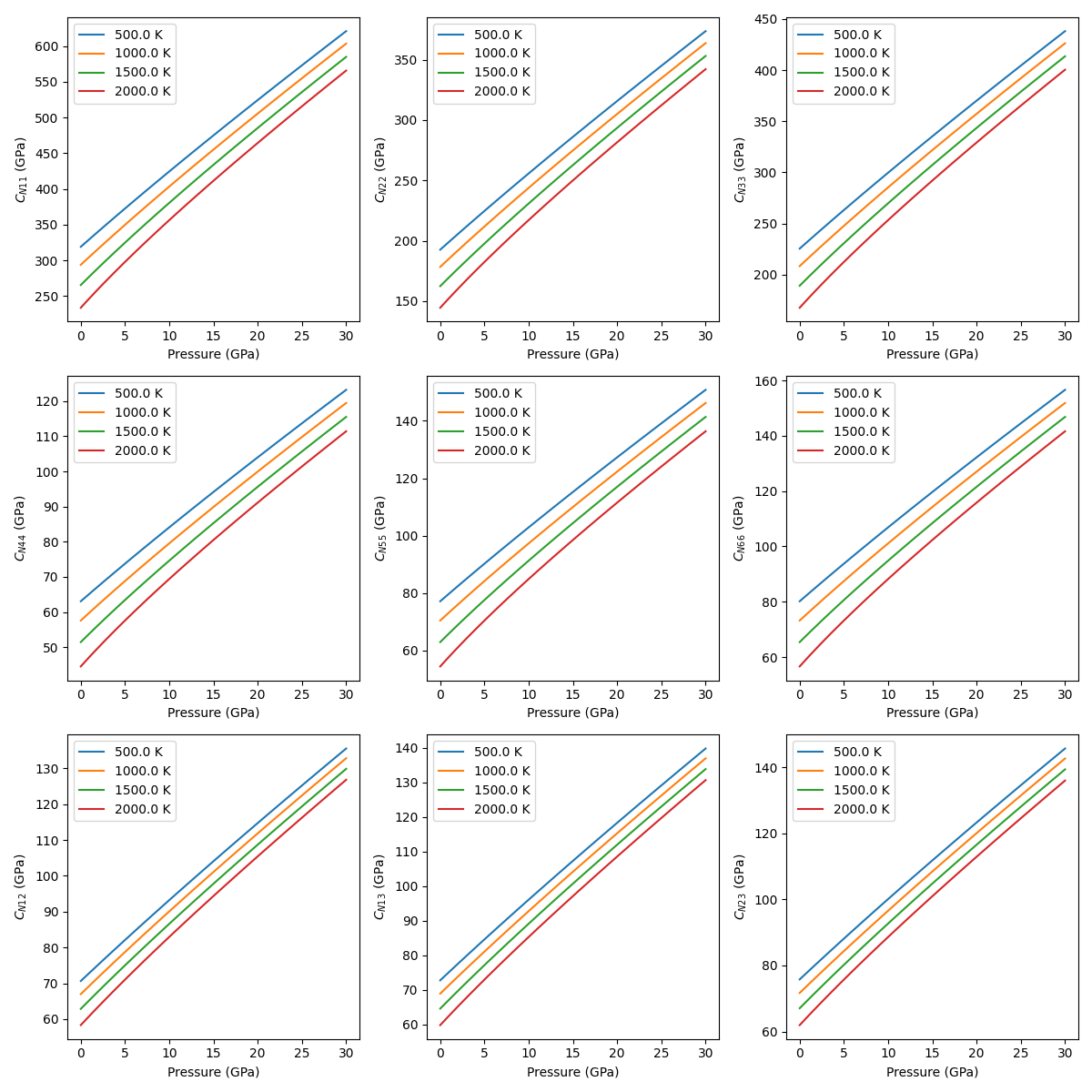### example_geotherms¶

This example shows each of the geotherms currently possible with BurnMan. These are:

1. Brown and Shankland, 1981 [BS81]

2. Anderson, 1982 [And82]

3. Watson and Baxter, 2007 [WB07]

4. linear extrapolation

5. Read in from file from user

6. Adiabatic from potential temperature and choice of mineral

Uses:

• burnman.geotherm.brown_shankland()

• burnman.geotherm.anderson()

• input geotherm file input_geotherm/example_geotherm.txt (optional)

• burnman.Composite for adiabat

Demonstrates:

• the available geotherms

Resulting figure: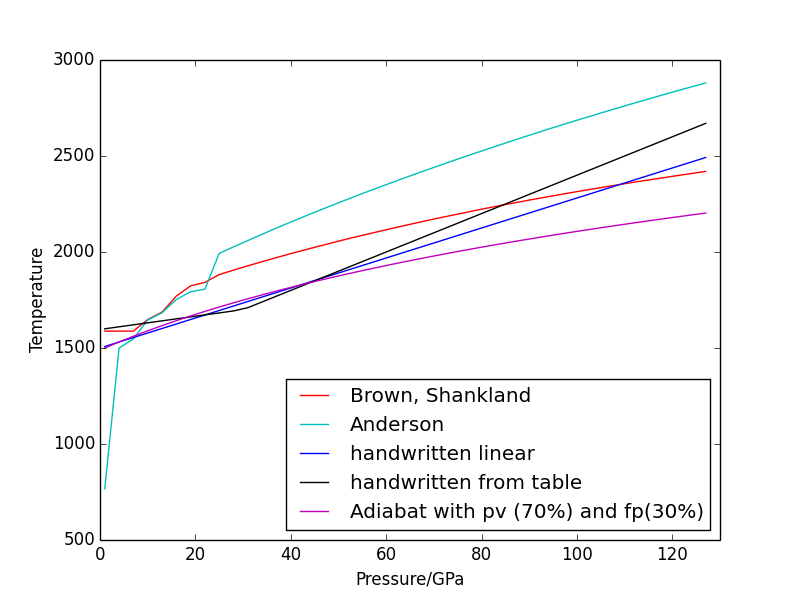### example_composition¶

This example script demonstrates the use of BurnMan’s Composition class.

Uses:

Demonstrates:

• Creating an instance of the Composition class with a molar or weight composition

• Printing weight, molar, atomic compositions

• Renormalizing compositions

• Modifying the independent set of components

• Modifying compositions by adding and removing components

## Simple Examples¶

The following is a list of simple examples:

### example_beginner¶

This example script is intended for absolute beginners to BurnMan. We cover importing BurnMan modules, creating a composite material, and calculating its seismic properties at lower mantle pressures and temperatures. Afterwards, we plot it against a 1D seismic model for visual comparison.

Uses:

Demonstrates:

• creating basic composites

• calculating thermoelastic properties

• seismic comparison

Resulting figure: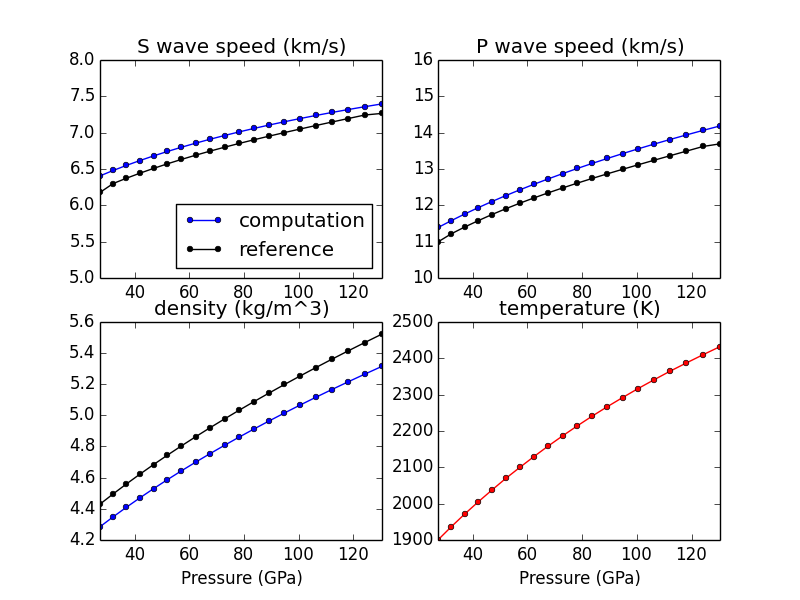### example_seismic¶

Shows the various ways to input seismic models ($$V_s, V_p, V_{\phi}, \rho$$) as a function of depth (or pressure) as well as different velocity model libraries available within Burnman:

1. PREM [DA81]

2. STW105 [KED08]

3. AK135 [KEB95]

4. IASP91 [KE91]

This example will first calculate or read in a seismic model and plot the model along the defined pressure range. The example also illustrates how to import a seismic model of your choice, here shown by importing AK135 [KEB95].

Uses:

Demonstrates:

• Utilization of library seismic models within BurnMan

• Input of user-defined seismic models

Resulting figures: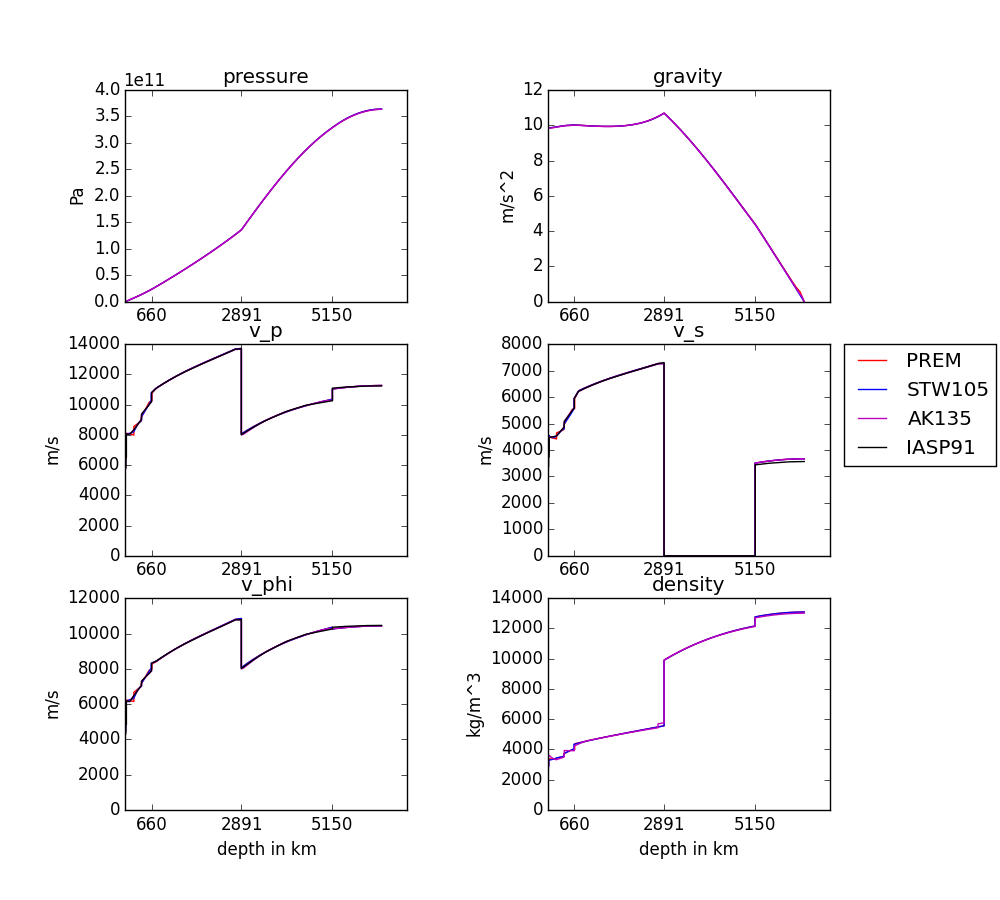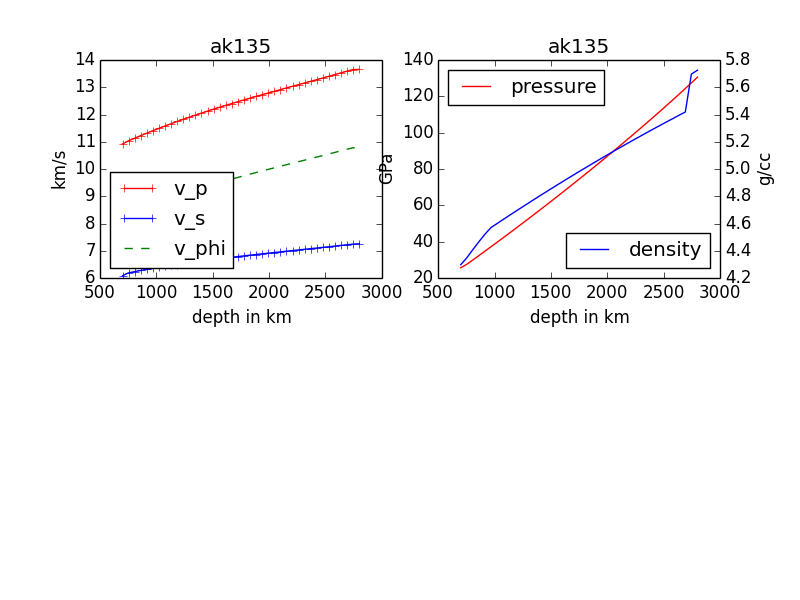### example_composite_seismic_velocities¶

This example shows how to create different minerals, how to compute seismic velocities, and how to compare them to a seismic reference model.

There are many different ways in BurnMan to combine minerals into a composition. Here we present a couple of examples:

1. Two minerals mixed in simple mole fractions. Can be chosen from the BurnMan libraries or from user defined minerals (see example_user_input_material)

2. Example with three minerals

3. Using preset solutions

To turn a method of mineral creation “on” the first if statement above the method must be set to True, with all others set to False.

Note: These minerals can include a spin transition in (Mg,Fe)O, see example_spintransition.py for explanation of how to implement this

Uses:

Demonstrates:

• Different ways to define a composite

• Using minerals and solutions

• Compare computations to seismic models

Resulting figure: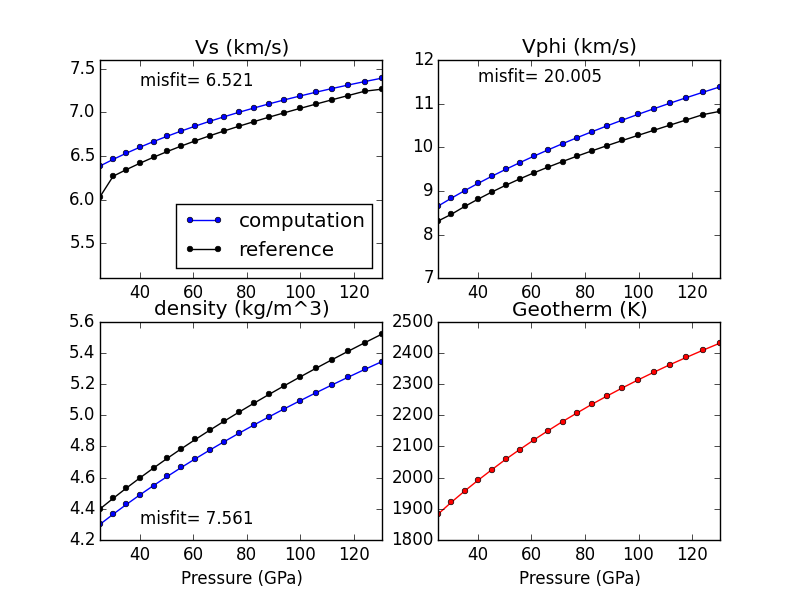### example_averaging¶

This example shows the effect of different averaging schemes. Currently four averaging schemes are available:

1. Voight-Reuss-Hill

2. Voight averaging

3. Reuss averaging

4. Hashin-Shtrikman averaging

See Journal of Geophysics and Space Physics for explanations of each averaging scheme.

Specifically uses:

Demonstrates:

• implemented averaging schemes

Resulting figure: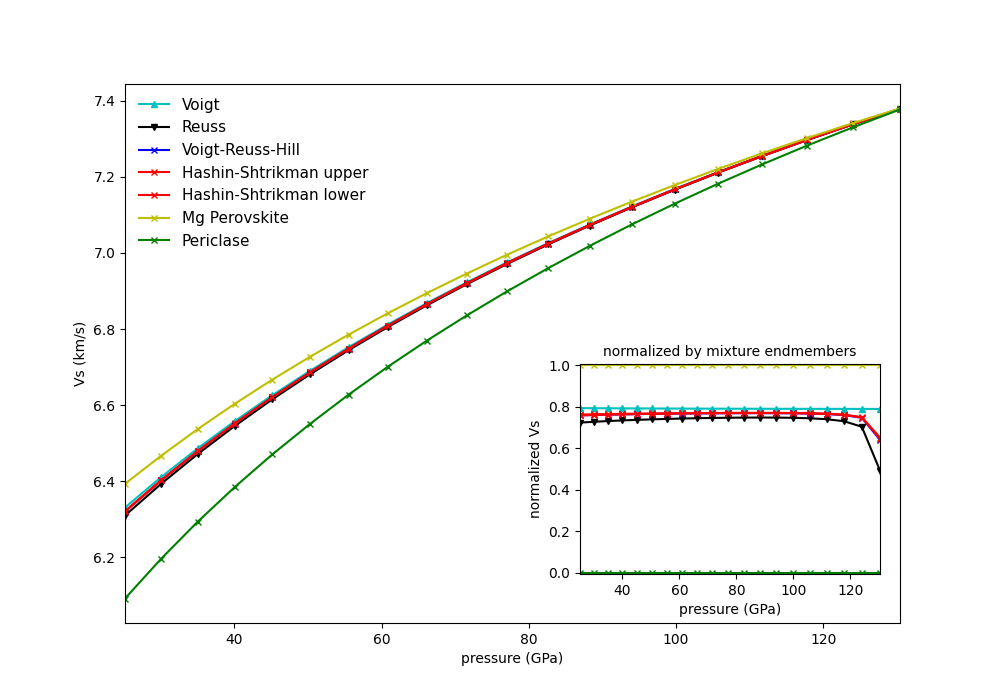### example_chemical_potentials¶

This example shows how to obtain chemical potentials and associated properties from an assemblage.

Demonstrates:

• How to calculate chemical potentials of an assemblage.

• How to compute fugacities and relative fugacities.

Resulting figure: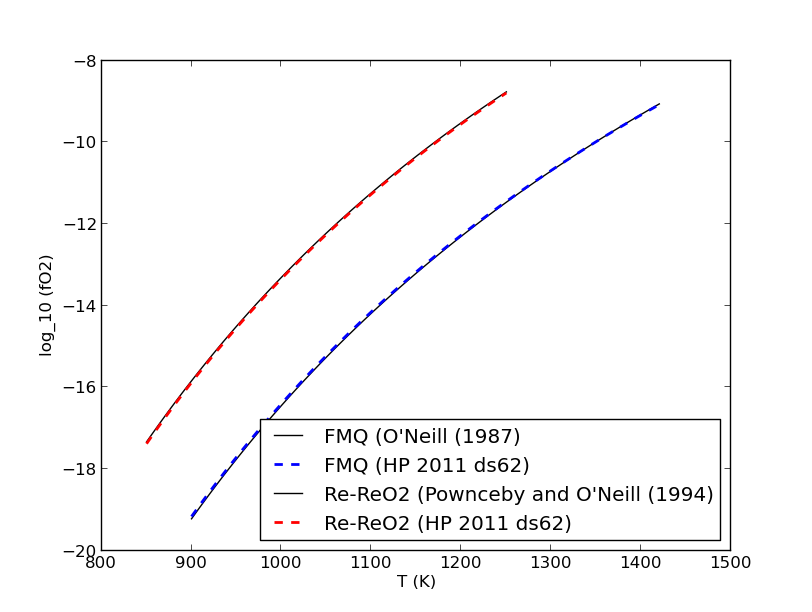### example_spintransition¶

This example shows the different minerals that are implemented with a spin transition. Minerals with spin transition are implemented by defining two separate minerals (one for the low and one for the high spin state). Then a third dynamic mineral is created that switches between the two previously defined minerals by comparing the current pressure to the transition pressure.

Specifically uses:

Demonstrates:

• implementation of spin transition in (Mg,Fe)O at user defined pressure

Resulting figure: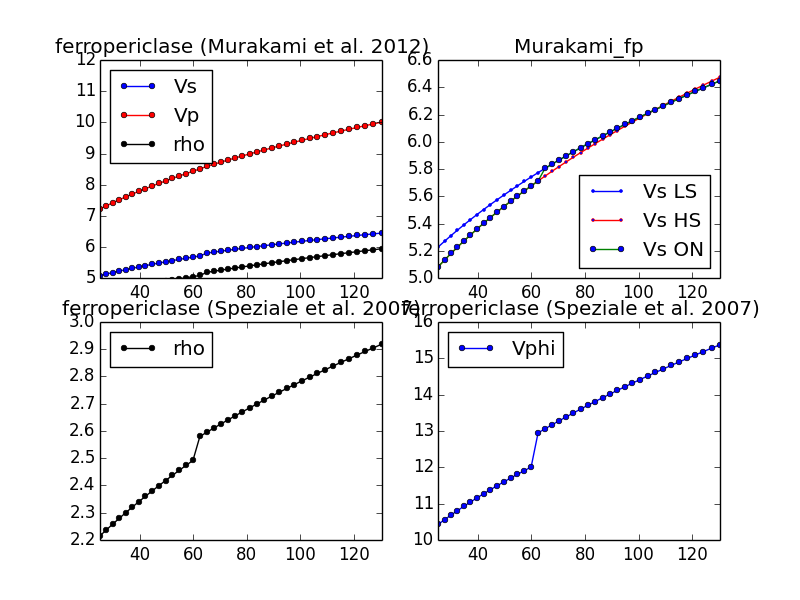### example_spintransition_thermal¶

This example illustrates how to create a non-ideal solution model for (Mg,FeHS,FeLS)O ferropericlase that has a gradual spin transition at finite temperature. First, we define the MgO endmember and two endmembers for the low and high spin states of FeO. Then we create a regular/symmetric solution that incorporates all three endmembers. The modified solution class contains a method called set_equilibrium_composition, which calculates the equilibrium proportions of the low and high spin phases at the desired bulk composition, pressure and temperature.

In this example, we neglect the elastic component of mixing. We also implicitly apply the Bragg-Williams approximation (i.e., we assume that there is no short-range order by only incorporating interactions that are a function of the average occupancy of species on each distinct site). Furthermore, the one site model [Mg,FeHS,FeLS]O explicitly precludes long range order.

Specifically uses:

Demonstrates:

• implementation of gradual spin transition in (Mg,Fe)O at a user-defined pressure and temperature

Resulting figure: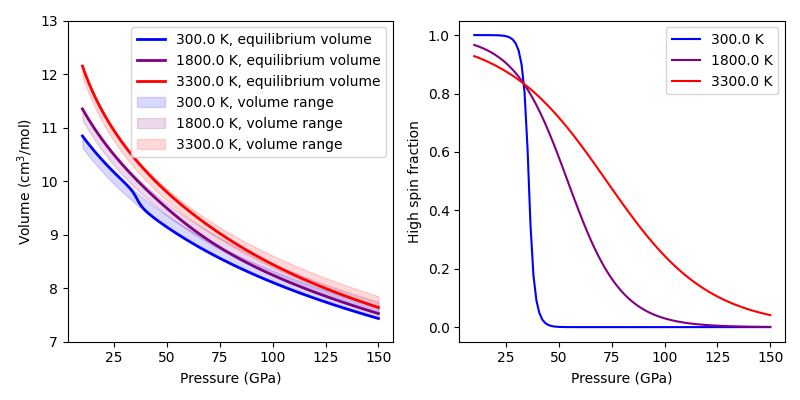### example_user_input_material¶

Shows user how to input a mineral of his/her choice without usint the library and which physical values need to be input for BurnMan to calculate $$V_P, V_\Phi, V_S$$ and density at depth.

Specifically uses:

Demonstrates:

• how to create your own minerals

### example_optimize_pv¶

Vary the amount perovskite vs. ferropericlase and compute the error in the seismic data against PREM. For more extensive comments on this setup, see tutorial/step_2.py

Uses:

Demonstrates:

• compare errors between models

• loops over models

Resulting figure: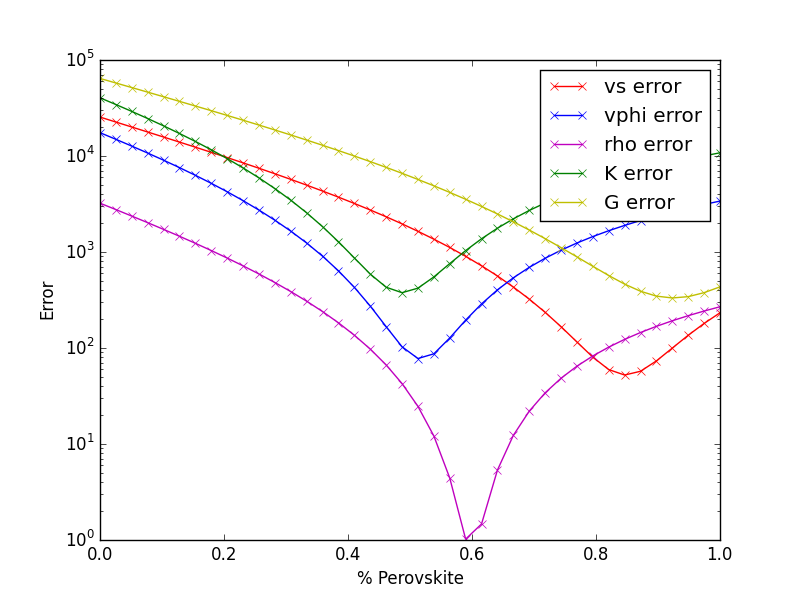### example_compare_all_methods¶

This example demonstrates how to call each of the individual calculation methodologies that exist within BurnMan. See below for current options. This example calculates seismic velocity profiles for the same set of minerals and a plot of $$V_s, V_\phi$$ and $$\rho$$ is produce for the user to compare each of the different methods.

Specifically uses:

Demonstrates:

• Each method for calculating velocity profiles currently included within BurnMan

Resulting figure: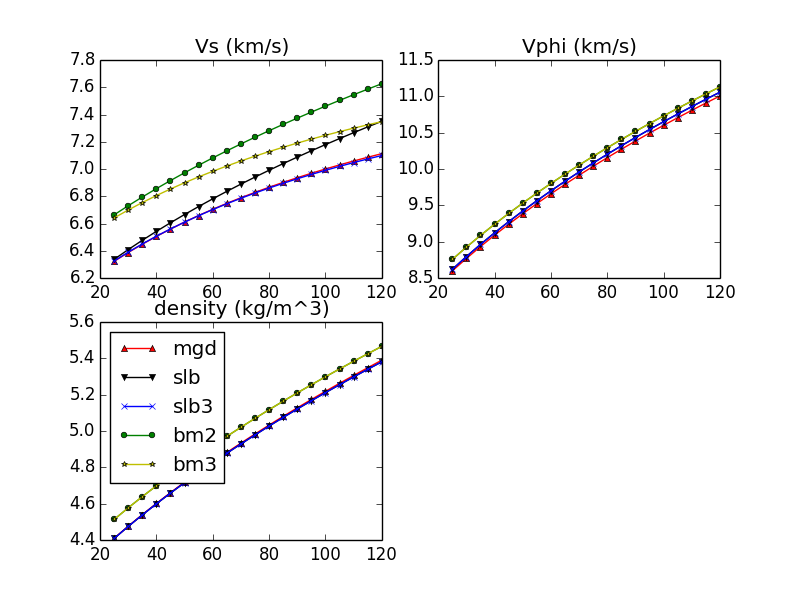### example_build_planet¶

For Earth we have well-constrained one-dimensional density models. This allows us to calculate pressure as a function of depth. Furthermore, petrologic data and assumptions regarding the convective state of the planet allow us to estimate the temperature.

For planets other than Earth we have much less information, and in particular we know almost nothing about the pressure and temperature in the interior. Instead, we tend to have measurements of things like mass, radius, and moment-of-inertia. We would like to be able to make a model of the planet’s interior that is consistent with those measurements.

However, there is a difficulty with this. In order to know the density of the planetary material, we need to know the pressure and temperature. In order to know the pressure, we need to know the gravity profile. And in order to the the gravity profile, we need to know the density. This is a nonlinear problem which requires us to iterate to find a self-consistent solution.

This example allows the user to define layers of planets of known outer radius and self- consistently solve for the density, pressure and gravity profiles. The calculation will iterate until the difference between central pressure calculations are less than 1e-5. The planet class in BurnMan (../burnman/planet.py) allows users to call multiple properties of the model planet after calculations, such as the mass of an individual layer, the total mass of the planet and the moment if inertia. See planets.py for information on each of the parameters which can be called.

Uses:

Demonstrates:

• setting up a planet

• computing its self-consistent state

• computing various parameters for the planet

• seismic comparison

Resulting figure: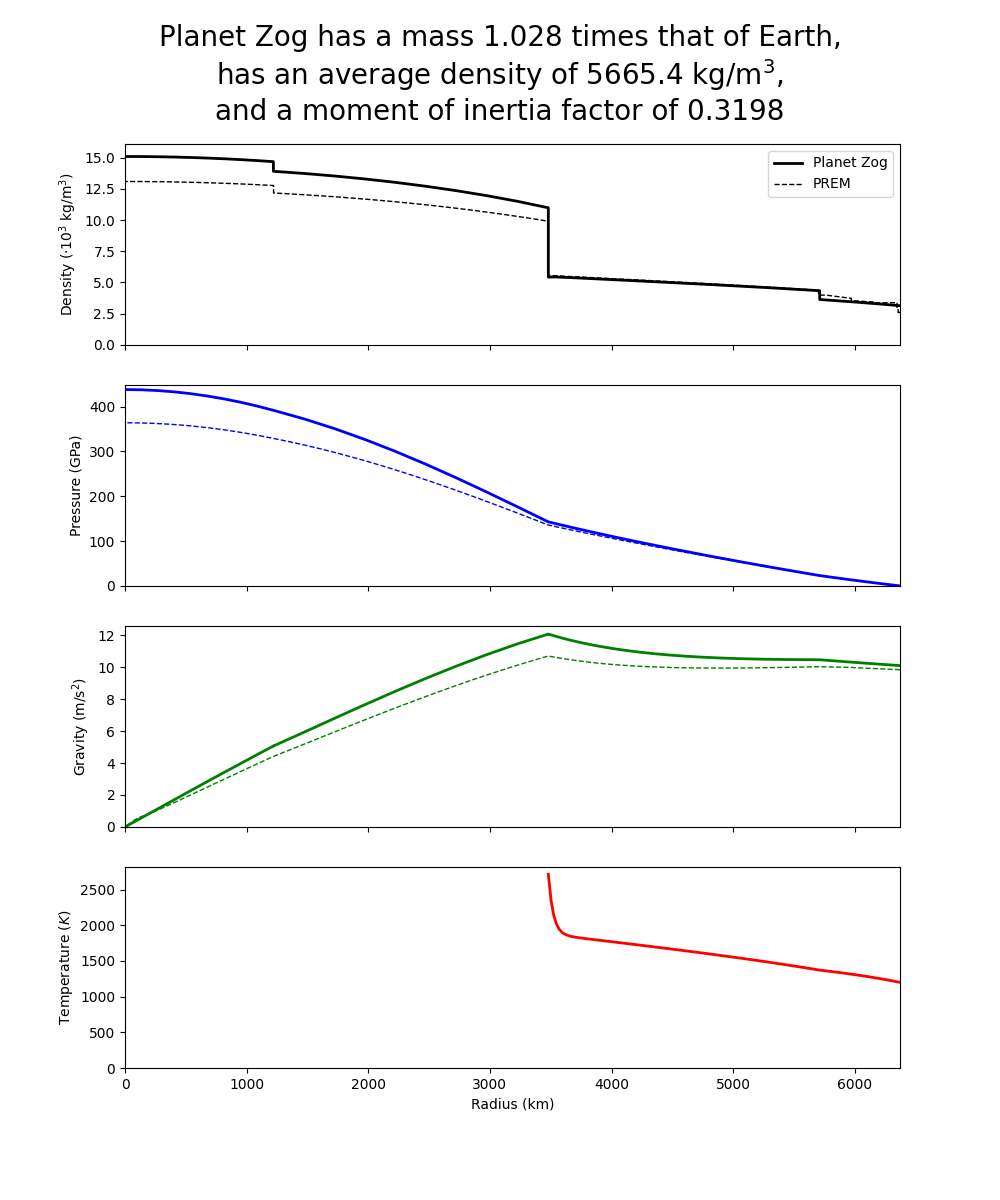### example_fit_composition¶

This example shows how to fit compositional data to a solution model, how to partition a bulk composition between phases of known composition, and how to assess goodness of fit.

Uses:

Demonstrates:

• Fitting compositional data to a solution model

• Partitioning of a bulk composition between phases of known composition

• Assessing goodness of fit.

Resulting figure: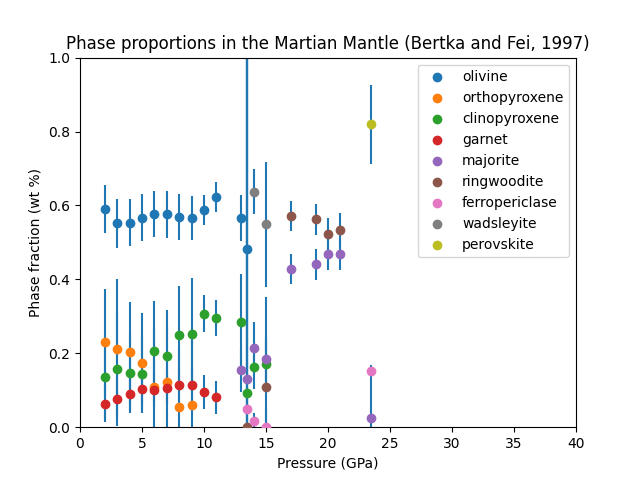### example_fit_data¶

This example demonstrates BurnMan’s functionality to fit various mineral physics data to an EoS of the user’s choice.

Please note also the separate file example_fit_eos.py, which can be viewed as a more advanced example in the same general field.

teaches: - least squares fitting

Resulting figures: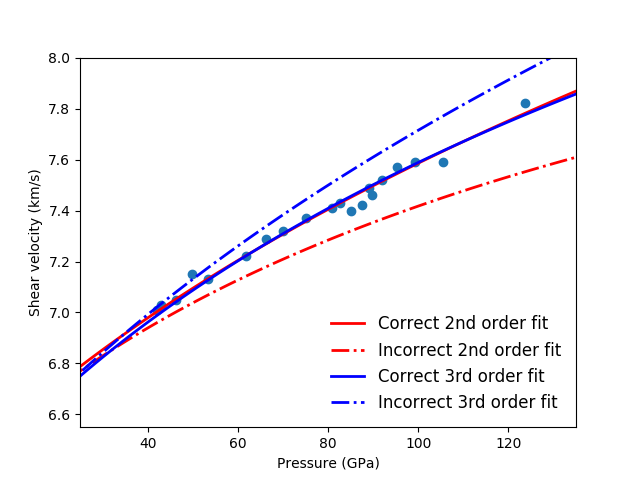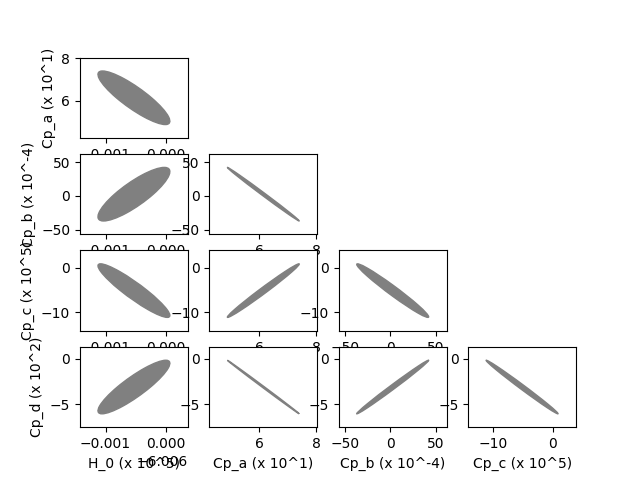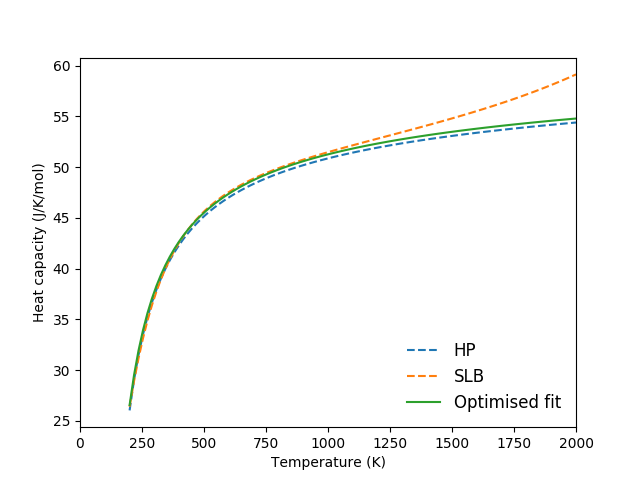### example_fit_eos¶

This example demonstrates BurnMan’s functionality to fit data to an EoS of the user’s choice.

The first example deals with simple PVT fitting. The second example illustrates how powerful it can be to provide non-PVT constraints to the same fitting problem.

teaches: - least squares fitting

Last seven resulting figures: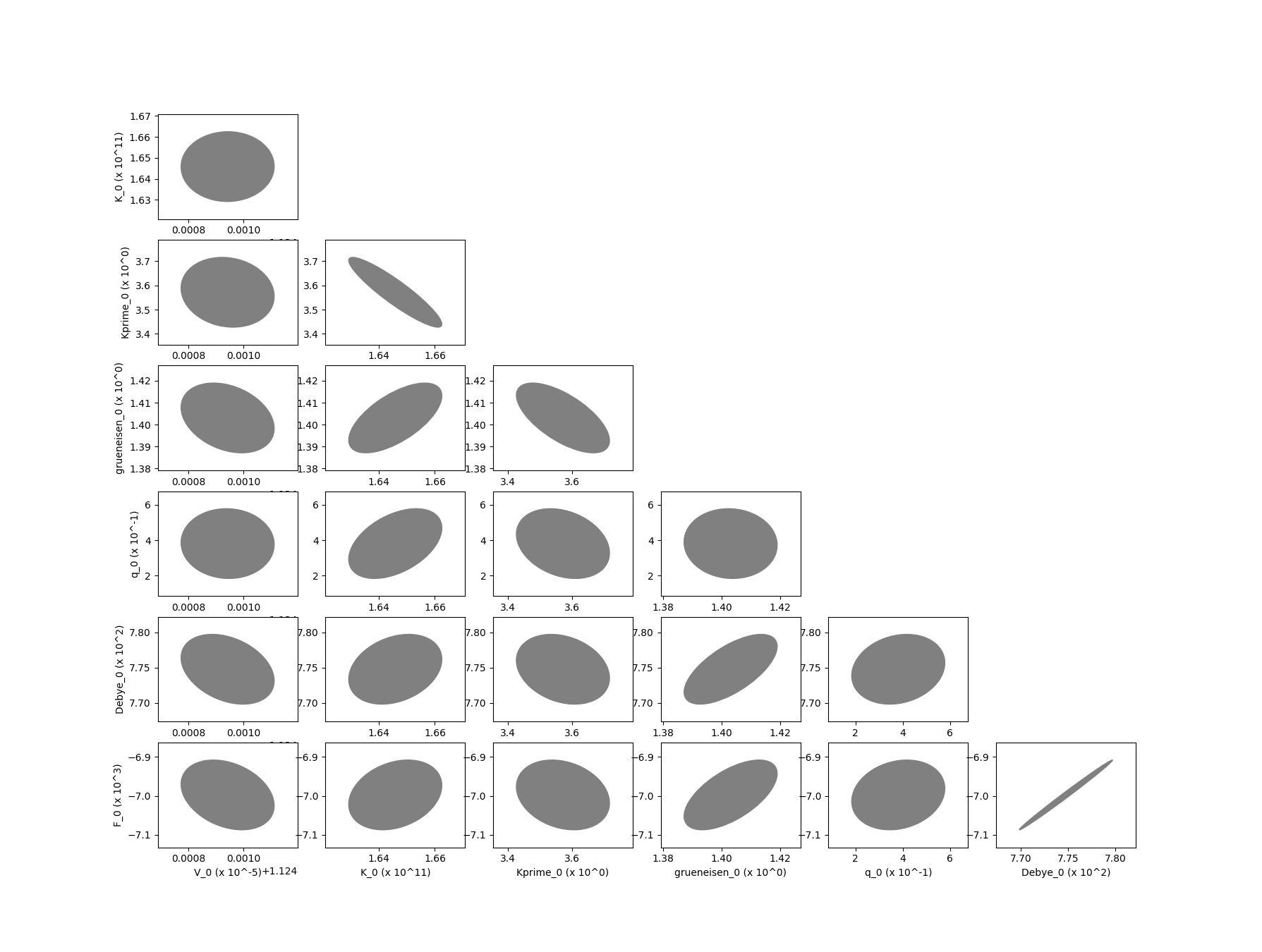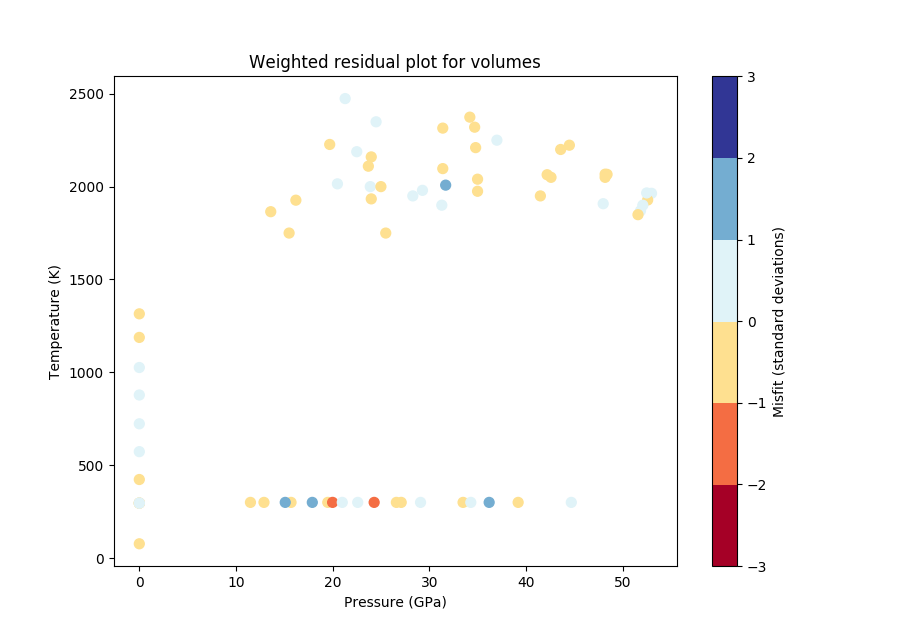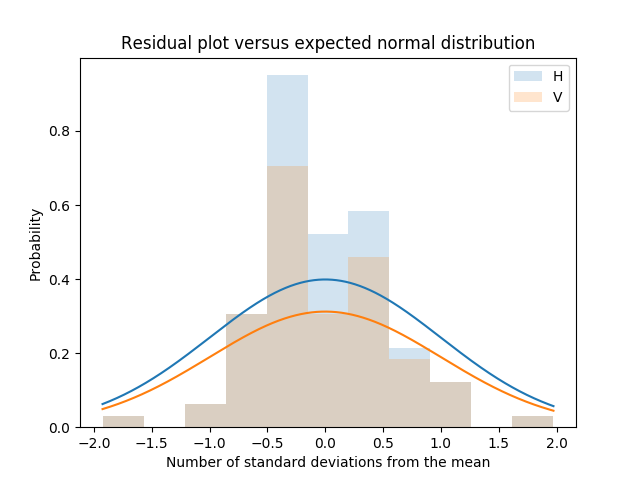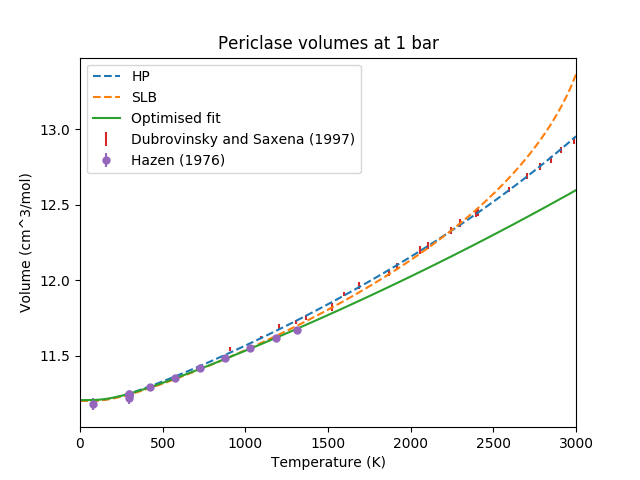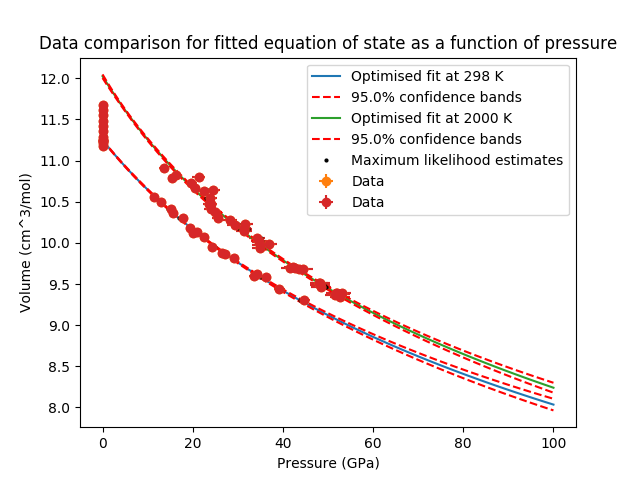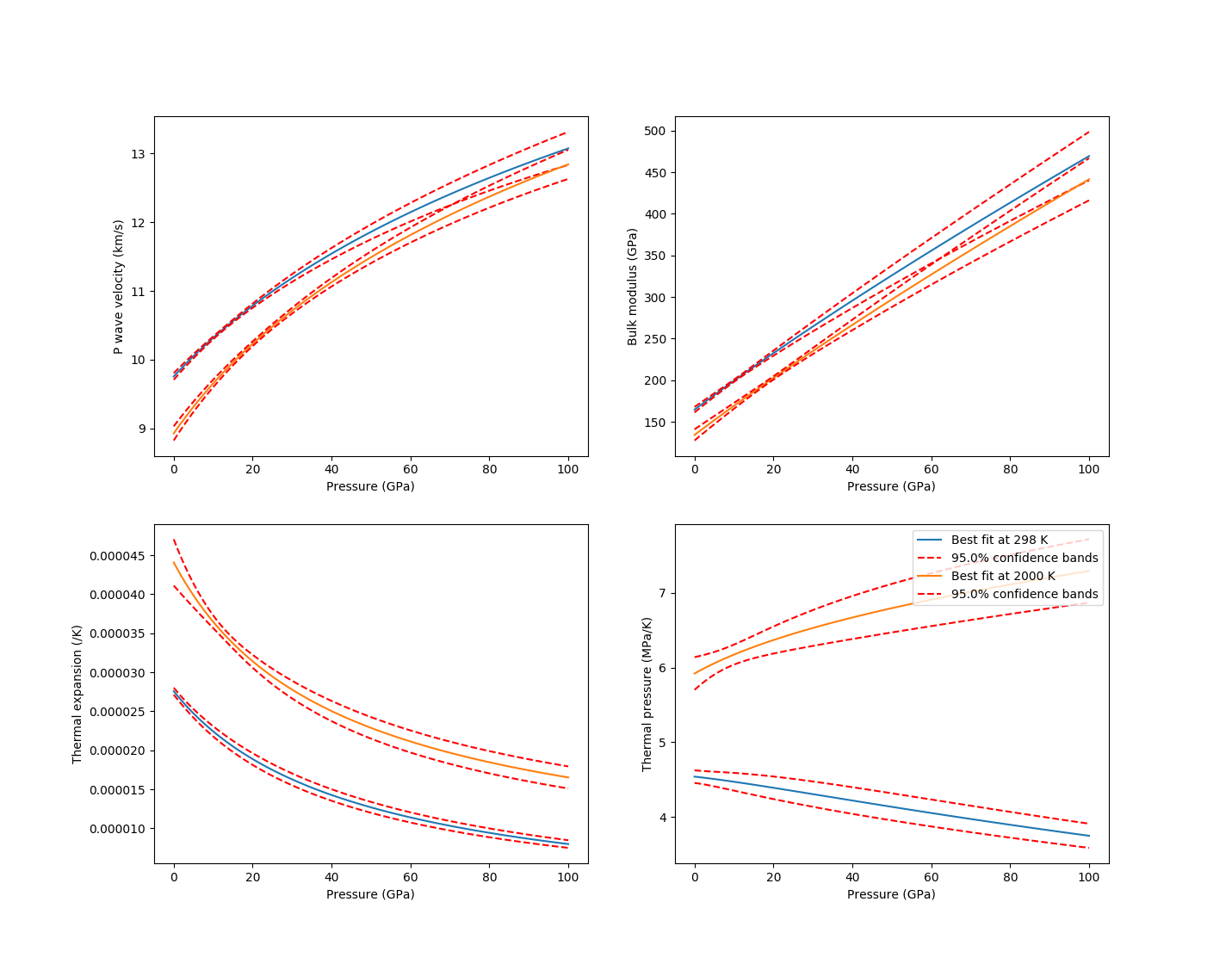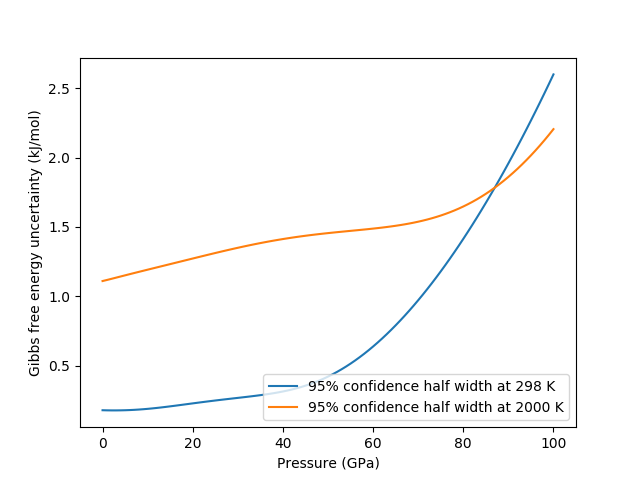### example_fit_solution¶

This example demonstrates how to fit parameters for solution models using a range of compositionally-variable experimental data.

The example in this file deals with finding optimized parameters for the forsterite-fayalite binary using a mixture of volume and seismic velocity data.

teaches: - least squares fitting for solution data

Resulting figures: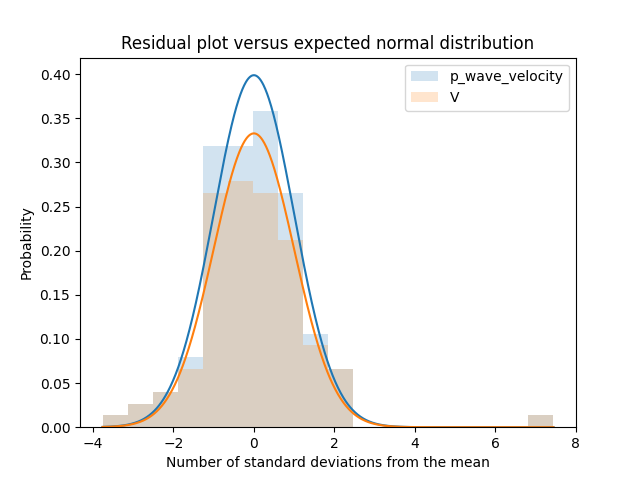Data residuals relative to the model fitted using all the provided data.¶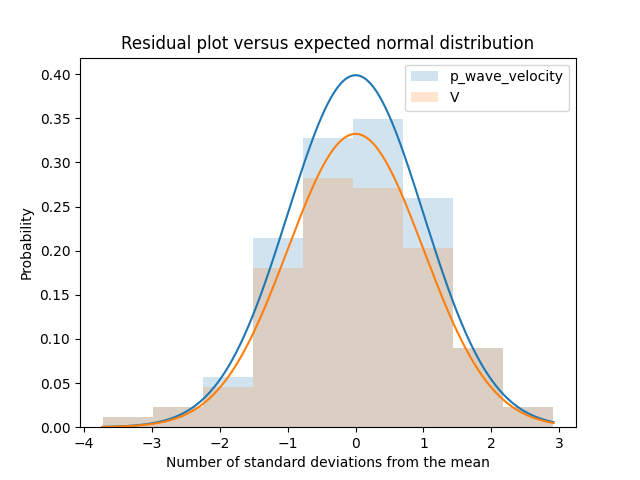Data residuals relative to the model fitted using the provided data after semi-automatic removal of spurious data.¶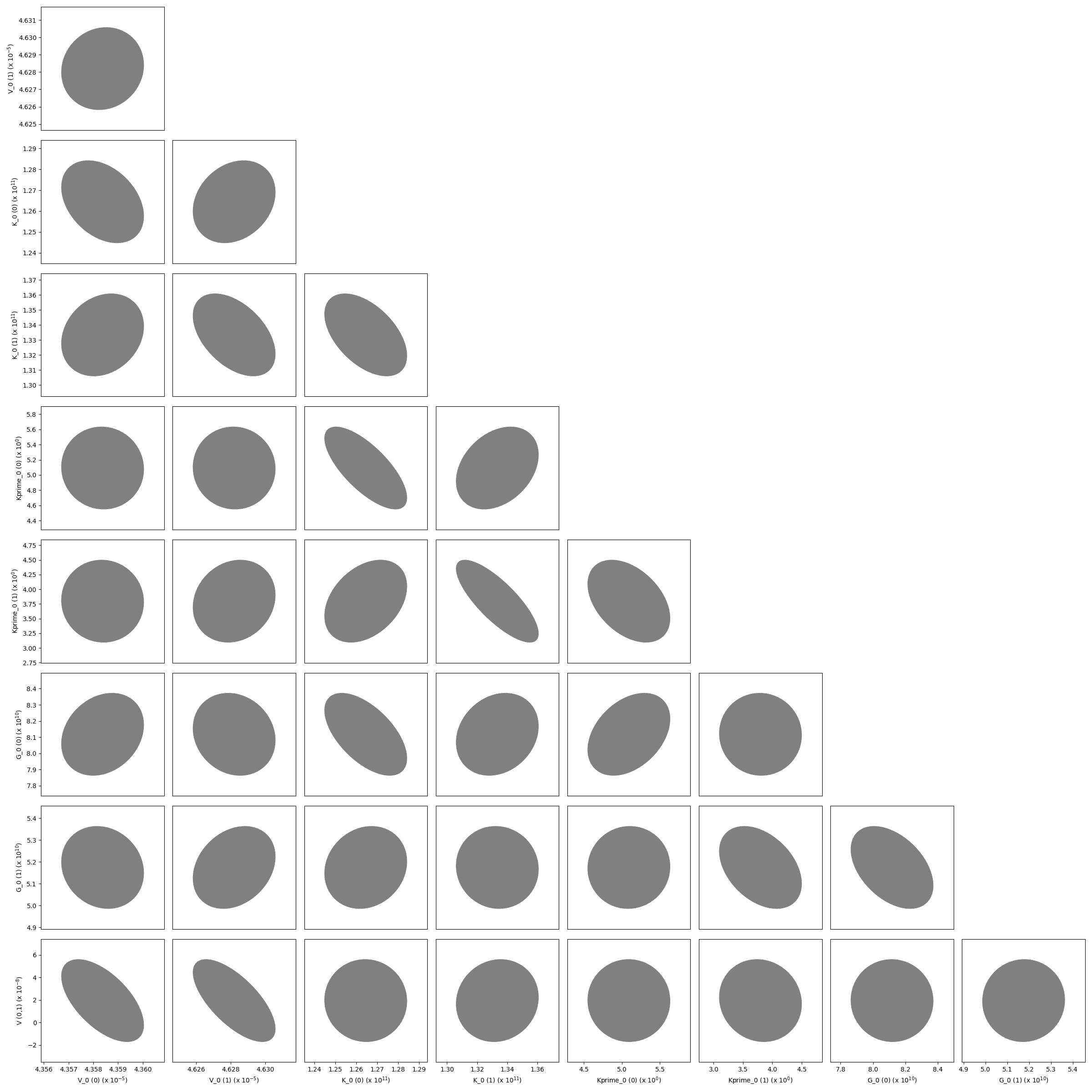The variance-covariance matrix of the optimized parameters shown as a corner plot.¶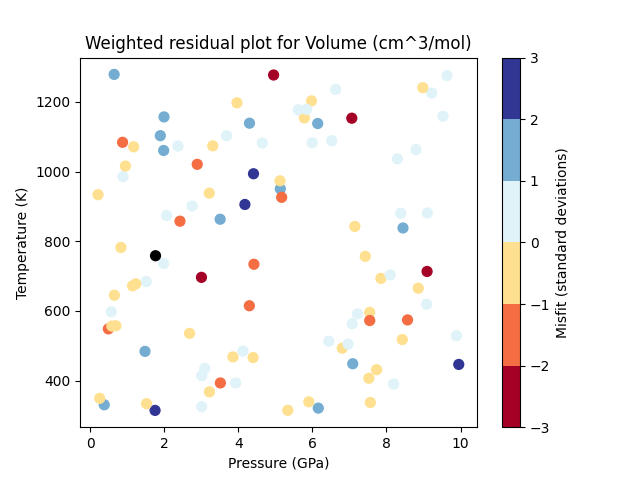A P-T plot showing the weighted residuals of each piece of volume data.¶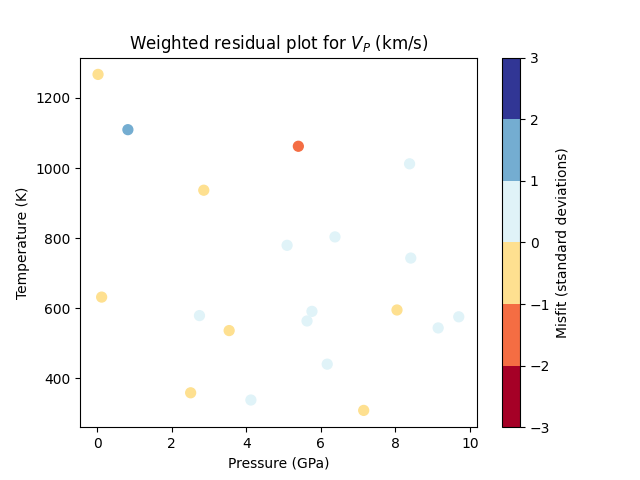A P-T plot showing the weighted residuals of each piece of P-wave velocity data.¶

### example_equilibrate¶

This example demonstrates how BurnMan may be used to calculate the equilibrium state for an assemblage of a fixed bulk composition given two constraints. Each constraint has the form [<constraint type>, <constraint>], where <constraint type> is one of the strings: P, T, S, V, X, PT_ellipse, phase_fraction, or phase_composition. The <constraint> object should either be a float or an array of floats for P, T, S, V (representing the desired pressure, temperature, entropy or volume of the material). If the constraint type is X (a generic constraint on the solution vector) then the constraint c is represented by the following equality: np.dot(c, x) - c. If the constraint type is PT_ellipse, the equality is given by norm(([P, T] - c)/c) - 1. The constraint_type phase_fraction assumes a tuple of the phase object (which must be one of the phases in the burnman.Composite) and a float or vector corresponding to the phase fractions. Finally, a phase_composition constraint has the format (site_names, n, d, v), where site names dictates the sites involved in the equality constraint. The equality constraint is given by n*x/d*x = v, where x are the site occupancies and n and d are fixed vectors of site coefficients. So, one could for example choose a constraint ([Mg_A, Fe_A], [1., 0.], [1., 1.], [0.5]) which would correspond to equal amounts Mg and Fe on the A site.

This script provides a number of examples, which can be turned on and off with a series of boolean variables. In order of complexity:

• run_aluminosilicates: Creates the classic aluminosilicate diagram involving univariate reactions between andalusite, sillimanite and kyanite.

• run_ordering: Calculates the state of order of Jennings and Holland (2015) orthopyroxene in the simple en-fs binary at 1 bar.

• run_gt_solvus: Demonstrates the shape of the pyrope-grossular solvus.

• run_fper_ol: Calculates the equilibrium Mg-Fe partitioning between ferropericlase and olivine.

• run_fixed_ol_composition: Calculates the composition of wadsleyite in equilibrium with olivine of a fixed composition at a fixed pressure.

• run_upper_mantle: Calculates the equilibrium compositions and phase proportions for an ol-opx-gt composite in an NCFMAS bulk composition.

• run_lower_mantle: Calculates temperatures and assemblage properties along an isentrope in the lower mantle. Includes calculations of the post-perovskite-in and bridgmanite-out lines.

• run_olivine_polymorphs: Produces a P-T pseudosection for a fo90 composition.

Uses:

Resulting figures:

### example_olivine_binary¶

This example demonstrates how BurnMan may be used to calculate the equilibrium binary phase diagram for the three olivine polymorphs (olivine, wadsleyite and ringwoodite).

The calculations use the equilibrate function. Unlike the examples in example_equilibrate.py, which are constrained to a fixed bulk composition, the bulk composition is allowed to vary along the vector [n_Mg - n_Fe].

Uses:

Resulting figures: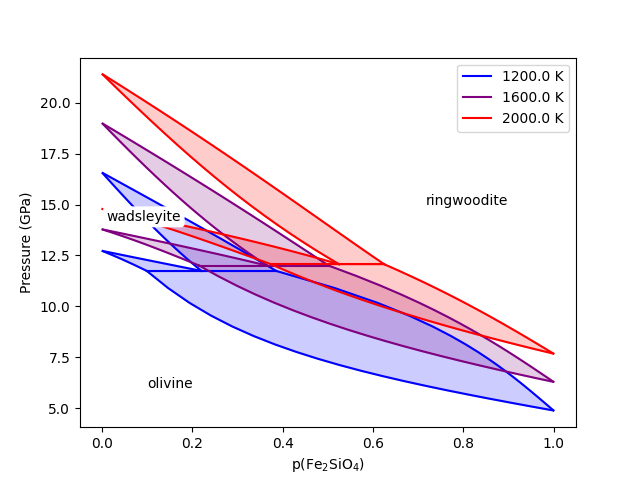The olivine polymorph binary phase diagram using the thermodynamic models of Stixrude and Lithgow-Bertelloni (2011).¶

## Reproducing Cottaar, Heister, Rose and Unterborn (2014)¶

In this section we include the scripts that were used for all computations and figures in the 2014 BurnMan paper: Cottaar, Heister, Rose & Unterborn (2014) [CHRU14]

### paper_averaging¶

This script reproduces [CHRU14], Figure 2.

This example shows the effect of different averaging schemes. Currently four averaging schemes are available: 1. Voight-Reuss-Hill 2. Voight averaging 3. Reuss averaging 4. Hashin-Shtrikman averaging

See for explanations of each averaging scheme.

requires: - geotherms - compute seismic velocities

teaches: - averaging

### paper_benchmark¶

This script reproduces the benchmark in [CHRU14], Figure 3.

### paper_fit_data¶

This script reproduces [CHRU14] Figure 4.

This example demonstrates BurnMan’s functionality to fit thermoelastic data to both 2nd and 3rd orders using the EoS of the user’s choice at 300 K. User’s must create a file with $$P, T$$ and $$V_s$$. See input_minphys/ for example input files.

requires: - compute seismic velocities

teaches: - averaging

contrib.CHRU2014.paper_fit_data.calc_shear_velocities(G_0, Gprime_0, mineral, pressures)[source]
contrib.CHRU2014.paper_fit_data.error(guess, test_mineral, pressures, obs_vs)[source]

### paper_incorrect_averaging¶

This script reproduces [CHRU14], Figure 5. Attempt to reproduce Figure 6.12 from [Mur13]

### paper_opt_pv¶

This script reproduces [CHRU14], Figure 6. Vary the amount perovskite vs. ferropericlase and compute the error in the seismic data against PREM.

requires: - creating minerals - compute seismic velocities - geotherms - seismic models - seismic comparison

teaches: - compare errors between models - loops over models

### paper_onefit¶

This script reproduces [CHRU14], Figure 7. It shows an example for a best fit for a pyrolitic model within mineralogical error bars.

### paper_uncertain¶

This script reproduces [CHRU14], Figure 8. It shows the sensitivity of the velocities to various mineralogical parameters.

## Misc or work in progress¶

### example_grid¶

This example shows how to evaluate seismic quantities on a $$P,T$$ grid.

### example_woutput¶

This example explains how to perform the basic i/o of BurnMan. A method of calculation is chosen, a composite mineral/material (see example_composition.py for explanation of this process) is created in the class “rock,” finally a geotherm is created and seismic velocities calculated.

Post-calculation, the results are written to a simple text file to plot/manipulate at the user’s whim.

requires: - creating minerals - compute seismic velocities - geotherms

teaches: - output computed seismic data to file